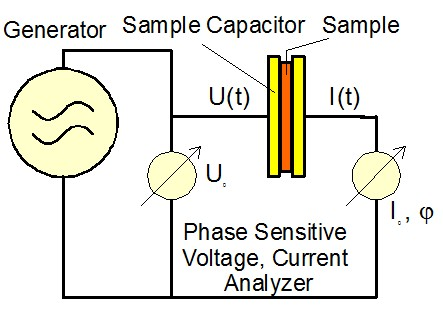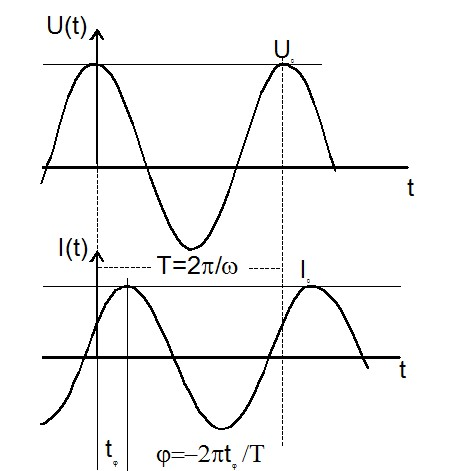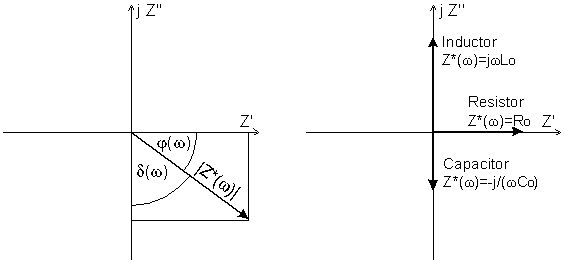## Impedance Measurement

The aim of impedance spectroscopy is to characterize the electrical properties of devices or materials by the impedance function Z*(ω). It is defined by the ratio of the voltage between two electrical ports of a sample object and the resulting current through the ports.Principle of impedance measurement According to this definition, impedance is similar to electric dc resistance which defines the ratio U/I where U and I are constant in time. In an impedance measurement, however, an alternating ac voltage U0 with a fixed frequency ω/2π is applied to the sample under test. U0 causes a current I0 at the same frequency in the sample. In addition, there will generally be a phase shift between current and voltage described by the phase angle φ.Fig. 1: Amplitude and phase relations between sample voltage and current for an impedance measurement.

The ratio of U0 and I0 and the phase angle j are determined by the electrical properties of the sample. For simple calculation and representation of the formulas, it is convenient to use complex notation.

 Voltage: U(t)*(ω) := U0cos(ωt) =Re[U*(ω) exp(jωt)] Current: I(t)*(ω) := I0cos(ωt+φ) =Re[I*(ω) exp(jωt+φ)] with U*=U'+jU'' =U0 and I*=I'+jI''; I0 = √[I'2+I''2]; tan(φ)=I''/I'

where U*, U', U'', U0, I*, I', I'', I0 and j all are frequency dependent. For a sample with linear electrical response, the ratio of U*(ω) and I*(ω) does not depend on the magnitude U0 of the applied voltage and the measurement result can be reduced to one complex function which can be defined by several basic representations. The most common one is the impedance

Z(ω)*=Z'(ω)+jZ''(ω)=U*(ω)/I*(ω)

Other often used representations are

 admittance: Y*(ω) := 1/Z*(ω) capacity: C*(ω) := -j/(ωZ*(ω)) inductance: L*(ω) := -jZ*(ω)/ω

These representations show the following properties.

For an ideal resistor or conductor with resistance R and conductance S=1/R, Z*(ω) = R, and Y*(ω) = S.

For an ideal capacitor with capacity C0, C*(ω) = C0. If a material is placed between two electrodes, C*(ω) is directly proportional to the complex material permittivity ε*(ω).

For an ideal inductor with inductance L0, L*(ω) = L0. If a material is placed into an inductive coil, L*(ω) is directly proportional to the complex material permeability µ*(ω).Fig. 2: Vector representation of Z*(ω) in the complex plane and vectors for ideal resistor, capacitor, inductor.

The used representation depends on the sample type and the personal preference of the researcher. In practice, samples are never ideal. Nevertheless, one generally selects a representation close to the electrical sample type. E.g. a mainly capacitive sample generally is represented in terms of C*(ω). Independent of the actual representation, one should keep in mind that they all contain the same information, i.e., the sample response current to an applied voltage.

Other often used terms are related the ratios of real to imaginary part of the above defined representations. These are
 loss factor tan(δ) := -Z'(ω)/Z''(ω) = -C''(ω)/C'(ω) and phase factor tan(φ) := 1/tan(δ).

The corresponding loss and phase angles are shown in the figure above.

Caseφtan(φ)δtan(δ)
Ideal resistor090°
Ideal capacitor-90°-∞0
Ideal inductor90°180°0

In impedance spectroscopy Z*(ω) is measured over a broad frequency range e.g. from mHz to several GHz in order to get as most information as possible.